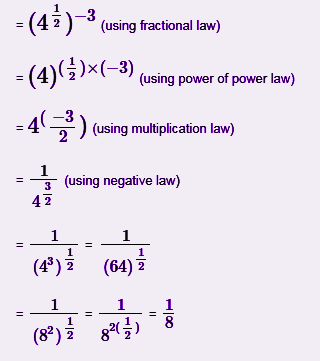# Exponent Rules

Exponent rules are the laws or basic principles based on which problems based on exponents are solved. The exponents are commonly seen not only in mathematics, but in every field. An exponent may be referred to a number or a variable raised to another number or variable. An exponential number can be written as an, where a = base and n = exponent. The laws of exponents are defined for different types of operations performed on exponents such as addition, multiplication and division.

When a number is multiplied by itself, the exponents are formed.

a x a = a2

a x a x a = a3

a x a x a x a = a4

a x a x a x a x a = a5

and so on.

It is denoted as, an, here, n is known as the exponent of a. It could be stated as “a raised to the power n” or “nth power of a”.

## Exponent Rules and Explanation

There are certain rules defined when we learn about exponent and powers. Let us suppose that p and q be the exponents, while x and y be the bases.

### Zero Rule

Zero exponent of a variable is one.

x0 = 1

### One Rule

One exponent of a variable is the variable itself

x1 = x

### Negative Rule

Negative exponent of a variable can be written as follows.

x-p = 1/xp

### Product Rules

(i) Product of exponents on same base results in sum of exponent on that base.

xp.xq = xp+q

(ii) Product of same exponent on different bases is equal to that exponent raised to whole product of the bases.

xp.yp = (xy)p

### Quotient Rules

(i) Division of two exponents having same base results in difference of exponents (numerator exponent – denominator exponent) raised to that base.

xp/xq = xp-q

(ii) Division of same exponent on different bases is written as the exponent raised to division of the bases.

xp/yp = (x/y)p

### Power Rules

Power rules are given below.

1. (xp)q = xpq
2. x1/p = p√x
3. xp/q = q√xp

## Exponent Rules Chart

 Zero Rule X0 = 1 One Rule X1 = X Negative Power Rule X-p = 1/Xp Product Rule Xp. Xq = Xp+q Division Rule Xp/Xq = Xp-q Power raised to power (Xp)q = Xpq Power raised to product of bases (XY)p =Xp.Yp Power raised to fraction (X/Y)p = Xp/Yp

### Exponent Rules Examples

Q.1: Solve the exponents 37 × 32

Solution: By the product rule of exponent;

37 × 32 = 37+2 = 39

Q.2: Solve the exponents 2-3 ×(−7)-3

Solution: By using power of same exponent law;

2-3 ×(−7)-3 = (2×(−7))-3 = (-14)-3

Q.3: Solve the exponent (32)5

Solution: By using product of product rule;

(32)5 = 32×5 = 310

Q.4: Solve the exponent 54/5

Solution: Using division rule;

54/5 = 54-1 = 53 = 125

Q.5: Simplify the exponent 2-5

Solution: Using the negative exponent rule;

2-5 = 1/25 = 1/32

Q.6: Simplify using laws of exponents (√4)-3

Solution: Using laws of fractional exponent;### Practice Problems

1. Solve and find the product of these two exponents 213 and 211
2. Solve and find the value of exponents (54)2
3. Solve and find the value of exponents {(4)×(6)}2